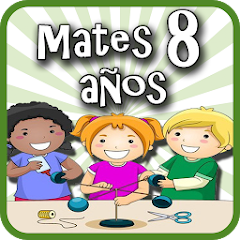# Matemáticas 8 años

100K+Everyone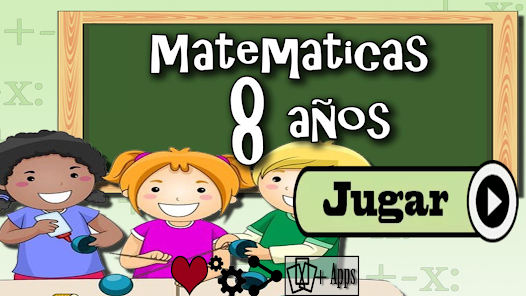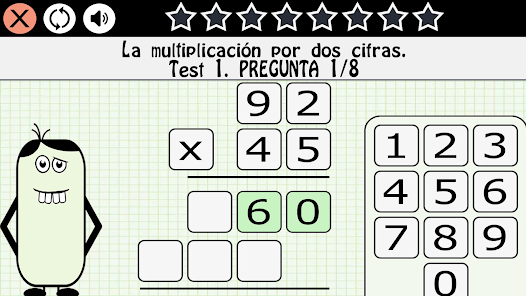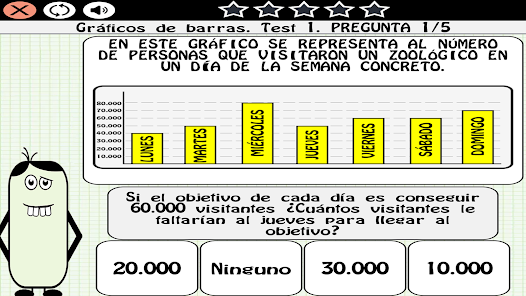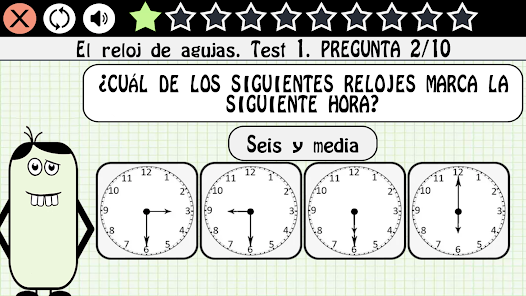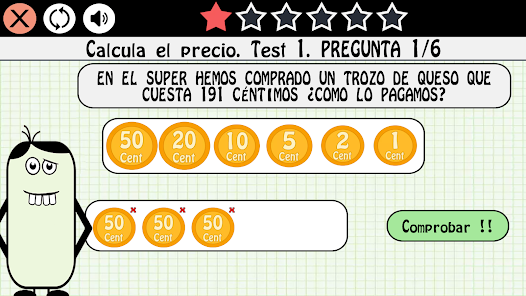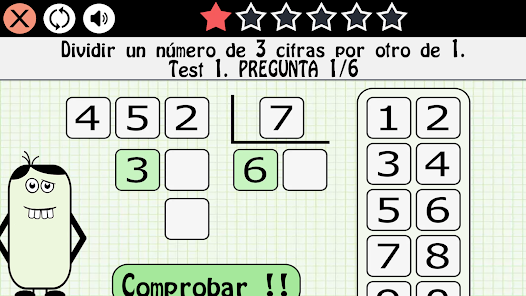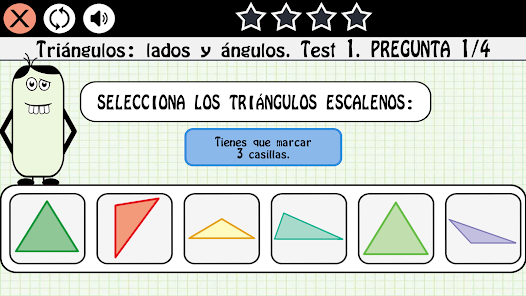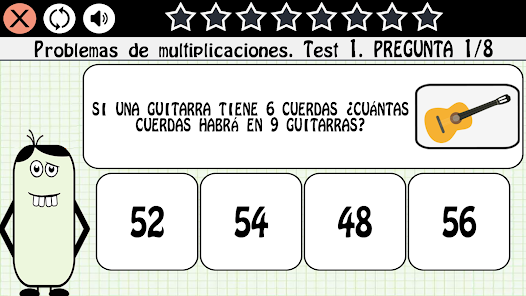Review the MATHEMATICS syllabus for 8-year-olds with our fun application, completing the topic map until you take the final exam.

- Totally free.
- Specially designed for children in third grade.
- More than 2000 questions.
- 45 topics: Numbers with 4 and 5 digits, Comparison of numbers, Ordering of numbers, The nearest hundred, The nearest thousand, Addition with carried, Subtraction with carried, Addition and subtraction problems with carried, Parentheses, Multiplication tables, Multiplication by one digit, Series of multiplication operations, Multiplication by two digits, Multiplication problems, Bar graphs, Exact division, Half of a number, Third of a number, Inexact division, Dividing a 3-digit number by a 1-digit number, Division problems, Lines and angles, Polygons, Circumference and circle, Symmetry, Day and hour, Minute and second, Clock hands , The digital clock, Calculate the price, The meter, Length measurement problems, The liter, The gram, Triangles, Polyhedrons, Prisms and pyramids.
- Final exam.
- Easy to play.
- Possibility to play without Internet.

Enjoy our application free of charge and reinforce your knowledge of mathematics for children of 8 years.
Updated on
Jan 4, 2023

## Data safety

Safety starts with understanding how developers collect and share your data. Data privacy and security practices may vary based on your use, region, and age. The developer provided this information and may update it over time.No data shared with third parties